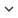ENGCongress
Congress 1058
Author/s
• Ismael Rodriguez-Fdez, Manuel Mucientes and Alberto Bugarin
DOI
Source
• 25th IEEE International Conference on Fuzzy Systems. Vancouver, Canada. 2016

# A Genetic Fuzzy System for Large-scale Regression

In genetic fuzzy systems (GFS) the size of the problem has a huge influence in the performance of the obtained models, since i) the fuzzy rule bases learned suffer from exponential rule explosion when the number of variables increases, and ii) the convergence time increases with the number of examples. In this paper we present S-FRULER, a scalable MapReduce version of FRULER which is a GFS that learns simple and linguistic TSK-1 knowledge bases for regression problems. S-FRULER obtains models with high accuracy and low complexity, whilst reducing the algorithm runtime. S-FRULER focuses on splitting the problem into smaller partitions and incorporates a feature selection process for reducing the number of variables used in each partition. Each partition is then solved independently using the FRULER algorithm. The Reduce function obtains linguistic TSK fuzzy rule bases from the information generated in each partition. S-FRULER has been validated in terms of scalability, precision and complexity using 10 large-scale datasets and has been compared with three state of the art GFSs. Experimental results show that S-FRULER scales well while achieving simple models with a linguistic approach and a precision comparable with approximative models.
Keywords: Genetic Fuzzy Systems, regression, instances selection, multi-granularity fuzzy discretization